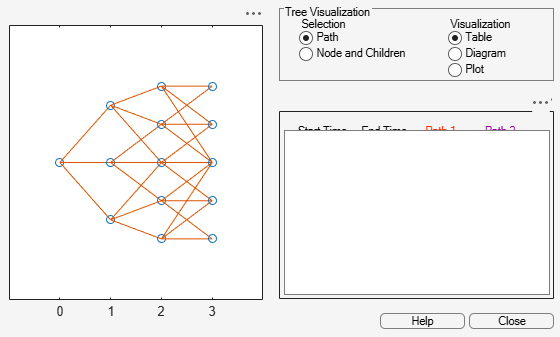# cvtree

Convert inverse-discount tree to interest-rate tree

## Syntax

``RateTree = cvtree(Tree)``

## Description

example

````RateTree = cvtree(Tree)` converts a tree structure using inverse-discount notation to a tree structure using rate notation for forward rates.```

## Examples

collapse all

Convert a Hull-White tree using inverse-discount notation to a Hull-White tree displaying interest-rate notation.

```load deriv.mat; HWTree```
```HWTree = struct with fields: FinObj: 'HWFwdTree' VolSpec: [1x1 struct] TimeSpec: [1x1 struct] RateSpec: [1x1 struct] tObs: [0 1 2 3] dObs: [731947 732313 732678 733043] CFlowT: {[4x1 double] [3x1 double] [2x1 double] } Probs: {[3x1 double] [3x3 double] [3x5 double]} Connect: { [2 3 4] [2 2 3 4 4]} FwdTree: {[1.0279] [1.0528 1.0356 1.0186] [1.0829 1.0652 1.0478 1.0306 1.0138] [1.0946 1.0767 1.0591 1.0418 1.0248]} ```
`HWTree.FwdTree{1}`
```ans = 1.0279 ```
`HWTree.FwdTree{2}`
```ans = 1×3 1.0528 1.0356 1.0186 ```

Use `treeviewer` to display the path of interest rates expressed in inverse-discount notation.

`treeviewer(HWTree)`Use `cvtree` to convert the inverse-discount notation to interest-rate notation.

`RTree = cvtree(HWTree)`
```RTree = struct with fields: FinObj: 'HWRateTree' VolSpec: [1x1 struct] TimeSpec: [1x1 struct] RateSpec: [1x1 struct] tObs: [0 1 2 3] dObs: [731947 732313 732678 733043] CFlowT: {[4x1 double] [3x1 double] [2x1 double] } Probs: {[3x1 double] [3x3 double] [3x5 double]} Connect: { [2 3 4] [2 2 3 4 4]} RateTree: {[0.0275] [0.0514 0.0349 0.0185] [0.0796 0.0632 0.0467 0.0302 0.0137] [0.0904 0.0739 0.0574 0.0409 0.0245]} ```
`RTree.RateTree{1}`
```ans = 0.0275 ```
`RTree.RateTree{2}`
```ans = 1×3 0.0514 0.0349 0.0185 ```

use `treeviewer` to display the converted tree, showing the path of interest rates expressed as forward rates.

`treeviewer(RTree)`## Input Arguments

collapse all

Tree structure, specified by Heath-Jarrow-Morton, Black-Derman-Toy, Hull-White, Black-Karasinski, or Cox-Ingersoll-Ross tree structure that uses inverse-discount notation for forward rates.

Data Types: `struct`

## Output Arguments

collapse all

Tree structure using rate notation for forward rates, returned as a tree structure.

## Version History

Introduced before R2006a